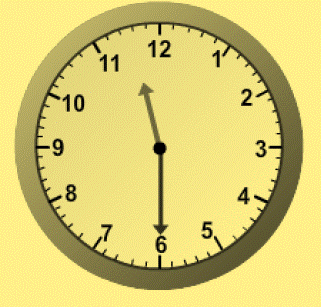1
visibility

What time does the clock below show?• A

11:30

• B

1:30

• C

12:30

• D

12:00

The correct answer is 11:30

Solution:

Consider the clock below:• The hour hand is between 11 and 12, so it is 11 hours.
• The minute hand is at 6. So, if we begin at 12 and count up by fives until we reach 6. It is 30 minutes.

So, the time shown by the clock is 11:30